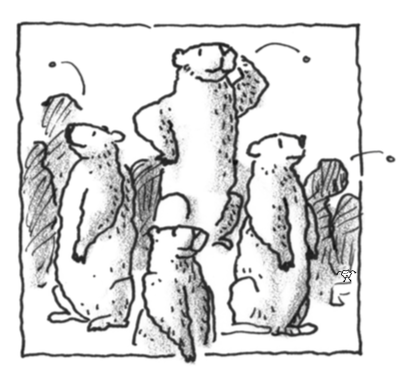### Home > APCALC > Chapter 12 > Lesson 12.2.2 > Problem12-80

12-80.

There are $50,000$ groundhogs on the Isle of Tork. Due to the isolation of the island (it is $1500$ miles from the nearest mainland) it had been spared the scourge of fleas. That was until a passing sailor on a round-the-world cruise stopped there to walk her dog. On its walk, the dog managed to pass fleas to four groundhogs. Although the dog is gone, the fleas have multiplied and they are spreading to the other groundhogs. In this case, the rate of infestation is proportional to the product of the number of groundhogs who do have fleas and the number who do not.1. Let $f$ represent the number of groundhogs who have fleas after $t$ days. Write a differential equation that models the spread of the fleas.

$\frac{df}{dt}=kf(50000-f)$

2. Write a general solution to the differential equation.

$\frac{df}{f(50000-f)}=k\cdot dt$

By partial fraction decomposition:

$\frac{1}{50000}\Big(\frac{1}{f}+\frac{1}{50000-f}\Big)df=k\cdot dt$

After integrating:

$\frac{1}{50000}\ln\Big|\frac{f}{50000-f}\Big|=kt+C$

$\frac{f}{50000-f}=e^{50000kt+C}=Ae^{50000kt}$

Keep rewriting to solve for $f$.

3. Recall that $f = 4$ when $t = 0$ because four groundhogs were initially infected. Suppose that after ten days, $2000$ groundhogs have fleas. Write an equation for $f$ in terms of $t$.

$4=\frac{50000A}{1+A}\text{ and }2000=\frac{50000Ae^{500000k}}{1+Ae^{500000k}}$